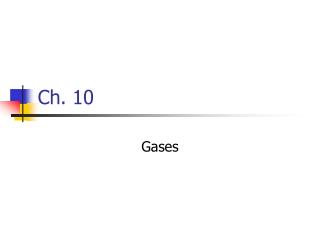DownloadDownload PresentationCh. 10

# Ch. 10

Télécharger la présentation## Ch. 10

- - - - - - - - - - - - - - - - - - - - - - - - - - - E N D - - - - - - - - - - - - - - - - - - - - - - - - - - -
##### Presentation Transcript

1. Ch. 10 Gases

2. Properties • Expand to fill their container • Highly compressible • Molecules are far apart

3. Pressure - conversions 1 atm = 760 mm Hg 1 atm = 760 torr 1 atm = 1.01325 x 105 Pa 1 atm = 101.325 kPa

4. The Gas Laws • Boyle’s: P and V • P1V1 = P2V2 • Inversely proportional • Weather balloons expand as they rise through the atmosphere • Breathing

5. Gas Laws • Charles’s Law: V and T • Directly proportional • Temperature in K!

6. Avogadro • 22.4 L of any gas at 0°C and 1 atm contains 6.022 x 1023 gas molecules (or 1 mol) • V = constant x n • Volume of a gas is directly proportional to the number of moles of that gas (if pressure and temp. are constant)

7. Ideal Gas Law • PV=nRT • STP = standard temperature and pressure (1 atm and 0°C)

8. Density and Molar Mass Calculations • M = dRT/P

9. Practice • 2NaN3 2Na + 3N2 • If an air bag has a volume of 36 L and is to be filled with nitrogen gas at a pressure of 1.15 atm at a temperature of 26.0°C, how many grams of NaN3 must be decomposed?

10. More than 1 Gas? • Dalton’s Law of Partial Pressures • Pt = P1 + P2 + P3 + …. • nt = n1 + n2 + n3 +…. • At constant temperature and volume, the total pressure is determined by the total number of moles present • Pt = (n1 + n2 + n3 +….) RT/V

11. Practice • A gaseous mixture made from 6.00 g O2 and 9.00 g CH4 is placed in a 15.0-L vessel at 0°C. What is the partial pressure of each gas, and what is the total pressure in the vessel?

12. Mole Fractions • Mole fraction – ratio of the number of moles of one component to the total number of moles in the mixture (part/whole) • Partial pressure of a gas in a mixture – mole fraction times the total pressure

13. Practice • Calculate the partial pressure of O2 in the mixture if the total pressure of the atmosphere is 745 torr. O2 is present at 18.0 mol percent.

14. Collecting Gases over Water

15. Practice • A sample of KClO3 is decomposed producing O2 gas that is collected over water. The volume of gas collected is 0.250 L at 26°C and 765 torr total pressure. How many grams of KClO3 were decomposed? Pressure of water vapor at 26°C is 25 torr.

16. KMT – Kinetic Molecular Theory – The ‘Why’ Behind Gas Behavior • Gases are always in motion (random) • Attractive and repulsive forces are negligible • Collisions are elastic – average kinetic energy of molecules does not change • At any given temperature, the molecules of all gases have the same kinetic energy

17. So, what…….. • If the temperature is doubled, the average kinetic energy is doubled (the individual molecules move at varying speeds)

18. The distribution of molecular speeds for N2 at three temperatures

19. Applications • Volume increase at constant temperature – longer distance to travel less collisions with the container walls decrease in pressure (Boyle’s Law) • Increase temperature at constant volume  increase kinetic energy increase collisions with container wall  increase pressure (Charles’s Law)

20. Practice • A sample of O2 gas initially at STP is compressed to a smaller volume at constant temperature. What effect does this change have on (a) the average kinetic energy of O2 molecules? (b) the average speed of O2 molecules? (c) the total number of collisions of O2 molecules with the container walls?

21. Effusion and Diffusion • Speed of a molecule is inversely proportional to its molar mass • Small = fast • Big = slow

22. Relationship between molar mass and molecular speed

23. The Process of Effusion – the escape of a gas molecule through a tiny hole into an evacuated space

24. Graham’s Law of Effusion • The effusion rate of a gas is inversely proportional to the square root of its molar mass • Lighter gases effuse more rapidly (b/c has more opportunities to get through the hole) – He balloon that deflates too quickly

25. Diffusion – spreading out of gas molecules • Why does it take a few minutes before you can smell the perfume that has been sprayed from across the room?

26. Real Gases –don’t always obey the ideal gas law • Do have finite volume (real gases tend to have larger volumes than predicted by the ideal gas law) • they do attract one another (causing a decrease in pressure) • **Deviate from ideal behavior at high pressures and low temperatures** • (Molecules of an ideal gas are assumed to occupy no space and have no attraction for one another)

27. Van der Waals • Introduced 2 constants, a and b, to account for the attractive forces felt by the “real” gases • nb = measure of volume occupied by gas molecules • n2a/V2 = attractive forces between molecules

28. Van der Waal’s constants for gas molecules The values of a and b generally increase with an increase in the mass of the molecule and an increase in the complexity of its structure; larger molecules tend to have greater intermolecular attractive forces